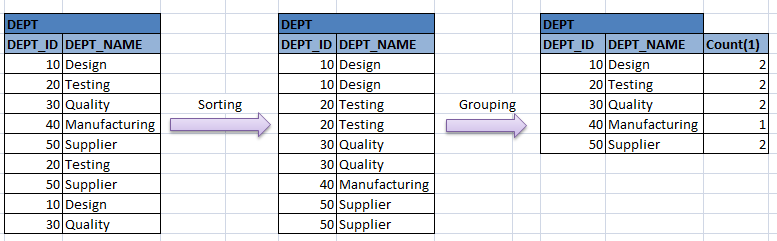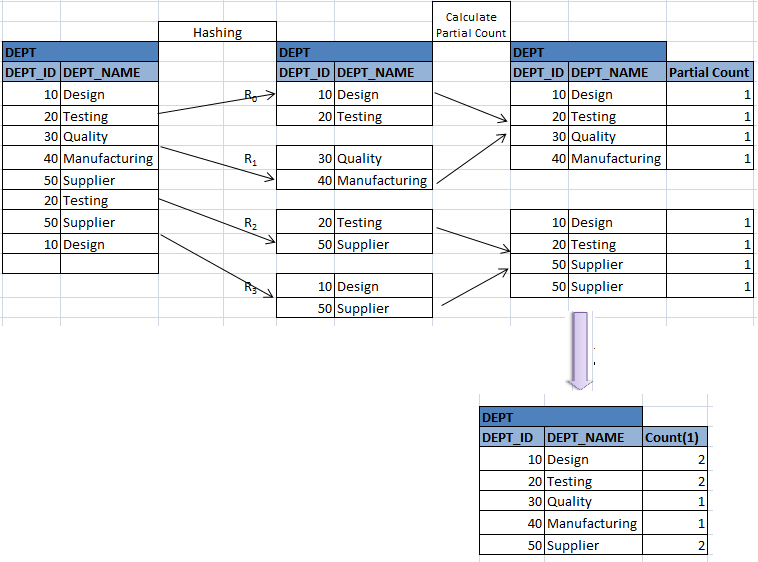# Grouping in DBMS

This is also implemented similar to duplicate elimination. Sorting or hashing techniques are used to get similar groups of records together. Then grouping functions like SUM, AVG etc are applied on it.

Suppose we want to see number duplicate records entered for each department in DEPT table. Since the table does not have any keys (hence it allowed entering duplicate records), the records are not sorted and are placed in the table as they are entered. In typical case, it has to pick one record and search the whole table for rest of the record to count the number for that record. Then it has to repeat the same step with rest of the records. This is a costly method of checking the counts. Hence what it does is, first sorts the records of DEPT table (either by sorting or hashing method). Then it counts the number of similar records and displays the results as below.

SELECT DEPT_ID, DEPT_NAME, COUNT (1) FROM DEPT GROUP BY DEPT_ID, DEPT_NAME;In hashing method, sorting can even optimized during run generation or merge passes. During run or merge passes, it can calculate the partial aggregate values in that block and can pass it to next merge pass.

For example, say we have one run and one merge pass in above case, and block size is 2 with one record each.We can see that here the task of grouping is distributed among the sorting steps and hence the cost of grouping at the final step is reduced drastically. Hence for better performance hashing method follows below steps while grouping.

• When MAX, MIN, SUM, COUNT are used in a query, partial value in each block is calculated and kept. It is passed to next level to calculate next partial value and so on.
• When AVG function is used in the query, SUM and COUNT are calculated in each step and at the end SUM is divided by the COUNT.
Translate »